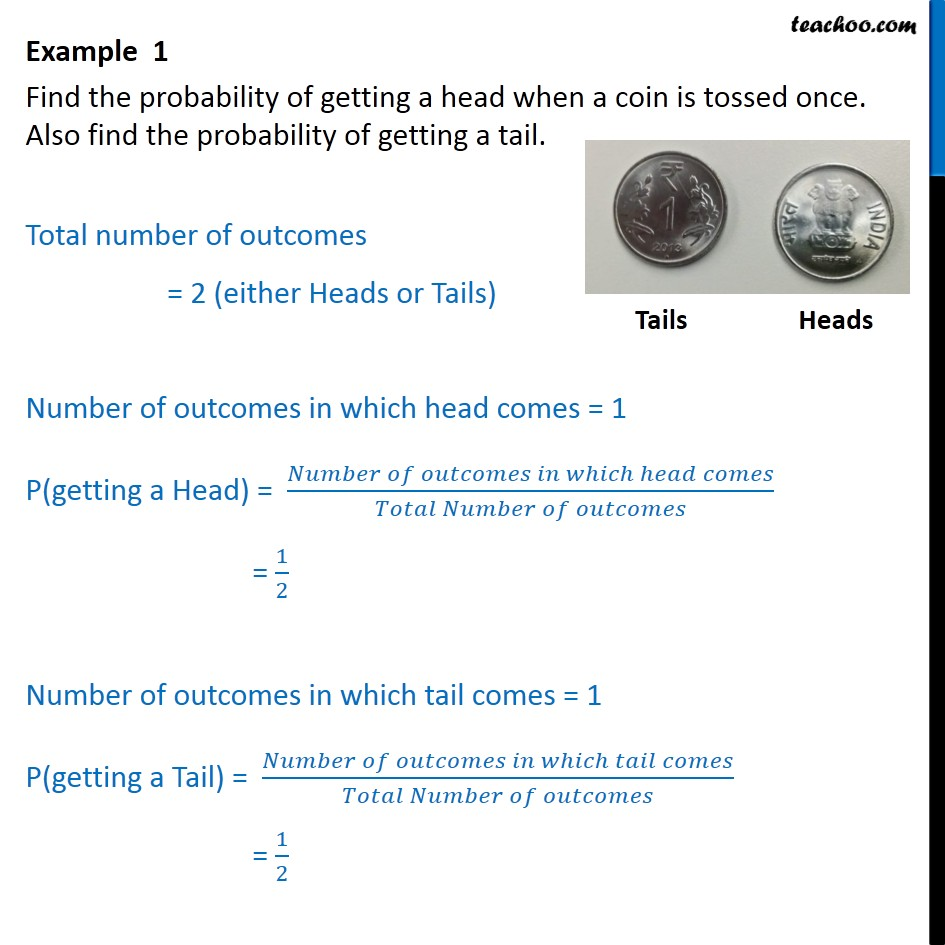Subscribe to our Youtube Channel - https://you.tube/teachoo

1. Chapter 15 Class 10 Probability
2. Concept wise
3. Coin

Transcript

Example 1 Find the probability of getting a head when a coin is tossed once. Also find the probability of getting a tail. Total number of outcomes = 2 (either Heads or Tails) Number of outcomes in which head comes = 1 P(getting a Head) = ( )/( ) = 1/2 Number of outcomes in which tail comes = 1 P(getting a Tail) = ( )/( ) = 1/2

Coin

About the AuthorDavneet Singh
Davneet Singh is a graduate from Indian Institute of Technology, Kanpur. He has been teaching from the past 9 years. He provides courses for Maths and Science at Teachoo.